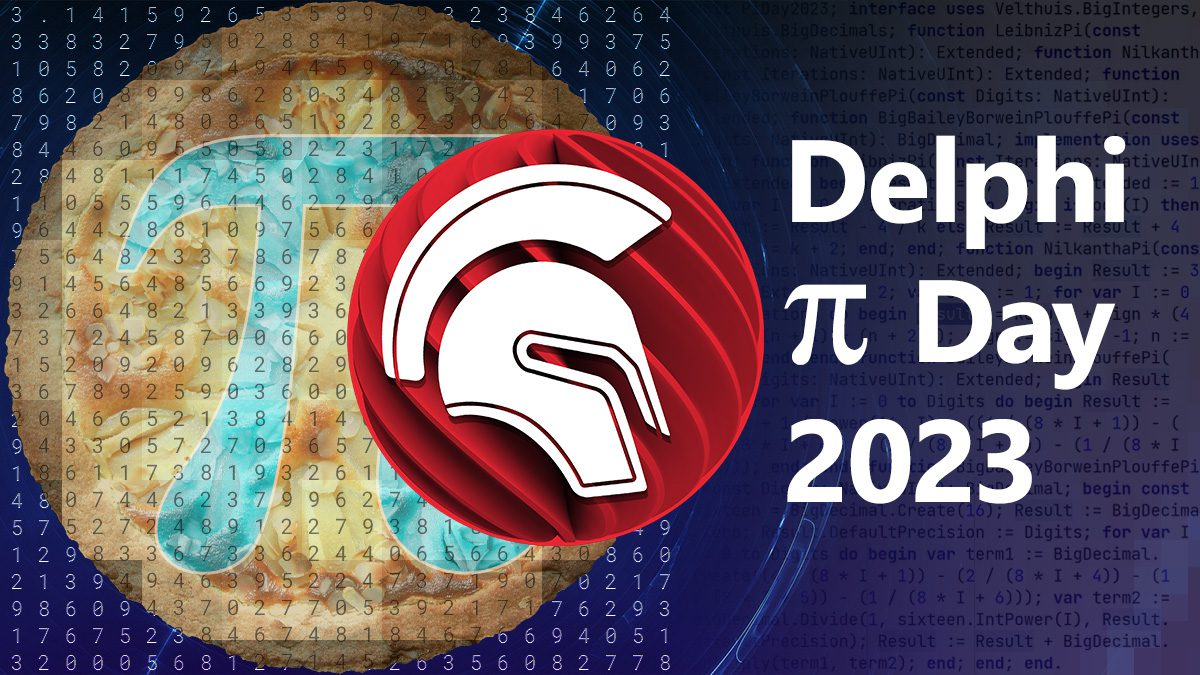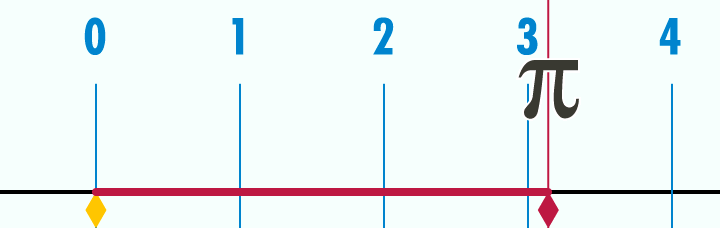Have an amazing solution built in RAD Studio? Let us know. Looking for discounts? Visit our Special Offers page!

# Calculating Pi in Delphi for Pi Day 2023In the United States today, March 14th is  π day (3rd month, 14th day, or 3/14). Traditions involve eating pie, the pastry, and calculating Pi, the irrational mathematical constant.Animation of the act of unrolling a circle’s circumference, illustrating the ratio π. Edited by John Reid [CC BY-SA 3.0] via Wikipedia

In Delphi, Pi is included as an intrinsic function. If you look in the System unit there are references, but no definition. It has a floating point value of 3.141592653589793238 (although you don’t usually see the last few digits). This is more accuracy than even NASA uses. But for theoretical mathematics and certain definitions of fun, we can do better.

As an irrational number, the decimal portion goes on forever without repeating. So when you calculate Pi you go through iterations improving your accuracy, or the number of digits precision. Google recently calculated 100 Trillion digits of Pi. It took 157 days, 23 hours, 31 minutes and 7.651 seconds on a 128 vCPUs with 864 GB RAM, 515 TB storage, and 82 PB of I/O. This was an opportunity to show off their cloud computing infrastructure. So the practical among us would just download the digits we need (as long as it is less than 100 trillion).

When it comes to calculating Pi there are a number of different algorithms. Many of us may have memorized a few digits, or some other approximation. But what if we want to calculate more? I poked around the web and even consulted Wolfram Alpha and ChatGPT. Here are a few examples I put together for you.

## Leibniz’s Formula for Pi

Named after Gottfried Leibniz, this formula comes from approximately the 14th century. I found a few examples in Delphi, and massaged them into the following implementation:

This ends up being rather slow and inaccurate. Running it for MaxInt iterations results in a value of 3.14159265312413, only accurate to 9 decimal places.

## Talking to Nilkantha

My notes say this is Nilkantha, but now I’m not sure. It was a bit more accurate than Leibniz, thus saving some time.

1000 iterations is accurate to 9 decimal places and 11,000 iterations is accurate to 12 places.

If someone recognizes this as a different algorithm, please let me know.

## The Bailey–Borwein–Plouffe Pi Forumula

This formula was discovered in 1995 by Simon Plouffe. The BBP formula gives rise to a spigot algorithm for computing the nth base-16 (hexadecimal) digit of π (and therefore also the 4nth binary digit of π). When I started trying to convert it to Delphi code I decided to give ChatGPT a shot, and it got me really close to working code. A little clean up gave me the following:

This one is very fast, and indicating 10 digits is more than enough.

## Enter Rudy Big Decimals

What if we want more than 10 digits of accuracy? Well, the late great Rudy Velthuis created a BigNumbers library which is available on GitHub. It includes BigIntegers, BigDecimals, and BigRationals, along with a number of other useful libraries. I converted the BBP algorithm to use BigDecimals and ended up with the following:

It is still very fast, and outputs very long numbers, but unfortunately I was still only able to get about 20 digits accurately.

## Conclusion

The pi built into Delphi is more than enough for most uses, but it is nice to look at the options for generating pi from scratch with Delphi. I’ve posted all my code on GitHub in a Gist, and will update it if I make any improvements based on more testing or feedback. Also, if you find yourself needing bigger Integers or Decimals, check out Rudy’s library.

[See all the code]